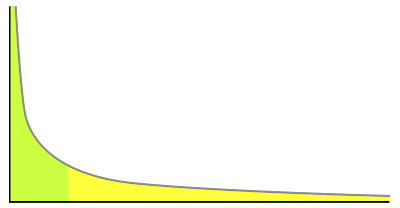# Thoughts on a Random Walk Model, from Victor NiederhofferI have always wondered to what extent a random walk with normally distributed steps would differ from a Pareto distribution with comparable means and third moments. I have never believed that stock prices are fractals or infinitely variance, or any way different from a shifting normal distribution or a mixture of same. The hazard rates of fall off for both distributions could be compared, and one would hypothesize that there would not be a observable difference.

## Ralph Vince writes:

Vic,

I have always agreed with precisely what you are saying. The "Pereto Explanation," does NOT explain market conditions changing; it is stationary. Clearly, those who posited that were naive when such might be better modelled by moving distributions (maybe even Cauchy distributions, where variance issues still persist).

However, when we speak of variance in returns, we must consider that the ntion of "infinite variance" is equally naive(!) as follows: What is varying is returns, specifically, today's price divided by some price in the past (e.g. yesterday for daily returns). Everyone seems to (conveniently) discount that these cannot go below zero. Thus, if returns were equivalently bound on the upside (at 200%) we could be certain that variance in returns was NOT infinite. Sine we cannot makes this assumption, we can only assume that variance can be infinite ONLY by upside moves of ever increasing maximum magnitude as we increase the window of time into the future.

We should be so lucky!

p.s. my own take on it is that we are dealing with moving distributions, likely Normal or at least where the variance is measurable and finite. The shape of the distribution, it;s moments, however, can move rapidly and without any warning whatsoever. price change, is just the single data point selected at that moment from such chrono-dynamic distributions, the real question pertaining to price change — and challenge — is to discern what that distributions is at the moment. THAT is the real price change, the solitary point witnessed, just a random manifestation of it.

## Steve Stigler writes:

On stock models: Some aspects of these models are driven by a wish for internal consistency, that the step distribution is the same (rescaled) for steps in minutes or hours, etc. And the steps are independent. These imply that the steps are "stable distributed", a class that includes normal and some heavy tail distributions. The reason is the central limit theorem (clt) - if the step for a minute was pareto with finite variance then the step for an hour would be the sum of 60 such and by the CLT approximately normal. If the step for a second is pareto, even more like a normal for an hour. So if you don't want a very complicated analysis the only choice with finite variance in normal, and if you take any other step dist for small steps the dist for large steps will be approx normal.